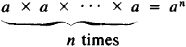# Involution

(redirected from Involutory)
Also found in: Dictionary, Thesaurus, Medical.

## involution

[‚in·və′lü·shən]
(biology)
A turning or rolling in.
(embryology)
Gastrulation by ingrowth of blastomeres around the dorsal lip.
(mathematics)
Any transformation that is its own inverse.
In particular, a correspondence between the points on a line that is its own inverse, given algebraically by x ′ = (ax + b)/(cx-a), where a 2+ bc ≠ 0.
A correspondence between the lines passing through a given point on a plane such that corresponding lines pass through corresponding points of an involution of points on a line.
(medicine)
The retrogressive change to their normal condition that organs undergo after fulfilling their functional purposes, as the uterus after pregnancy.
The period of regression or the processes of decline or decay which occur in the human constitution after middle life.

## Involution

(raising to a power), an algebraic operation consisting in repeating a quantity a n number of times:The number a is the base of the power, n is the exponent, and an is the power. For example, 3x3x3x3 = 34 = 81. The second power of a number is called its square and the third power, its cube.

## Involution

(regression), in biology, a reduction or loss, in the process of evolution, of certain organs, and a simplification of their organization and functions (for example, various degrees of intestinal reduction in certain parasitic worms and arthropods). Degenerative changes in protozoans and bacteria caused by unfavorable conditions in the habitat are also called involutional. In medicine, the term sometimes refers to atrophy of organs and tissues, such as in aging.

References in periodicals archive ?
We proved that if u is closed under an involutory antimorphism [PSI] and [D.sup.[PSI]] (u) is finite, then u is a morphic image of a word v with [D.sup.[PHI]](v) =0 for some involutory antimorphism [PHI].
Note that if G = {Id, [PSI]} where [PSI] is an involutory antimorphism, then [Pal.sup.[PSI]](w) is in one-to-one correspondence with the set [Pal.sup.G](w) (the only difference is that the latter is a set of orbits instead of factors).
where [G.sup.(2)] denotes the set of all involutory antimorphisms from G, see Pelantova and Starosta (2013).

Site: Follow: Share:
Open / Close